Courses

# Test: Electronic Devices - 1

## 10 Questions MCQ Test RRB JE for Electronics & Communication Engineering | Test: Electronic Devices - 1

Description
This mock test of Test: Electronic Devices - 1 for Electronics and Communication Engineering (ECE) helps you for every Electronics and Communication Engineering (ECE) entrance exam. This contains 10 Multiple Choice Questions for Electronics and Communication Engineering (ECE) Test: Electronic Devices - 1 (mcq) to study with solutions a complete question bank. The solved questions answers in this Test: Electronic Devices - 1 quiz give you a good mix of easy questions and tough questions. Electronics and Communication Engineering (ECE) students definitely take this Test: Electronic Devices - 1 exercise for a better result in the exam. You can find other Test: Electronic Devices - 1 extra questions, long questions & short questions for Electronics and Communication Engineering (ECE) on EduRev as well by searching above.
QUESTION: 1

### In a semiconductor which of the following carrier can contribute to the current?

Solution:

In a semiconductor, two types of charges are there by which the flow of the current takes place. So, both the holes and electrons take part in the flow of the current.

QUESTION: 2

### The Fermi level in a semiconductor bar should:

Solution:

Under equilibrium:

• The fermi level across the entire material will be sum and does not vary with distance.
• If there is any disturbance in the material, like junction contact, injection of impurities at any point, the charge carriers redistribute themselves such that the fermi-potential is same in entire material.

Under non-equilibrium:

• The fermi level is uneven with gradient of charge distribution across distance, in material.
• It can be studied using quasi-fermi states, related to charge distribution.
*Answer can only contain numeric values
QUESTION: 3

### Hole mobility in Ge at room temperature is 1900 cm2/V-sec. The diffusion coefficient is ________cm2/sec. (Write answer to one decimal point.)  (Take kT = 25 mV)

Solution:

Using the relation, D = 0.025 × 1900 cm2/sec
= 47.5 cm2/sec

QUESTION: 4

In the band diagram of a semiconductor, the fermi level is 0.3 eV above the intrinsic level. The energy level E in the diagram represents:

Solution: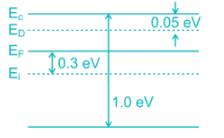• Given that fermi level is 0.3 eV above the intrinsic level, hence the type of semiconductor is n-type.
• In n-type the donor level is just below the conduction band and above the fermi level.
QUESTION: 5

The Difference between the donor energy level and fermi level in a n-type semiconductor in where 25% of the atoms are ionised at 300 k is:

Solution:

As 25% of donor atoms are ionised, the occupation probability of donor level is 0.75.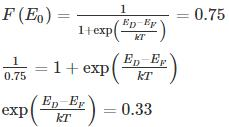ED - EF = -0.028 eV

*Answer can only contain numeric values
QUESTION: 6

A semiconductor with intrinsic carrier concentration 1 × 1010 cm-3 at 300°K has both valence and conduction band effective densities of states NC and NV equal to 1019 cm-3. The band gap Eg is _____ eV.

Solution:

The intrinsic concentration is related to bandgap by: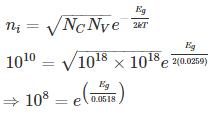Eg = 0.0518 ln (108) eV

= 0.954 eV

*Answer can only contain numeric values
QUESTION: 7

In a very long p-type Si bar with doping concentration Na = 1017 cm-3, excess holes are injected such that excessive concentration of holes at x = 0 is 5 × 1016 cm-3. The hole concentration at x = 1 μm is _____ × 1017 cm-3. Take μp = 500 cm2/v-s and recombination time constant τ = 10-8 s, kT = 0.0259 eV

Solution:

Diffusion constant: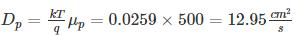Diffusion length: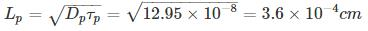• Hole concentration at any distance x is sum of equillibrium and excess hole concentration excess hole concentration varies with the distance x as Δp e-x/Lp
• p = p + Δp e-x/Lp
Where po = Doping concentration
Δp = extra hole concentration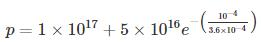= (1+.379) x 1017
1.379 × 1017 cm-3
QUESTION: 8

In an intrinsic semiconductor, the Fermi energy level EF doesn’t lie in the middle of the band gap cause:

Solution:
• In an intrinsic semi-conductor the Fermi energy: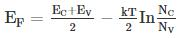Where NC and NV are density of state in valence and conduction bond respectively.
• Since NC ∝ mn, effective mass of electron and Nv ∝ mp, effective mass of hole. Since mn and mp are not equal: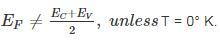QUESTION: 9

A silicon bar is doped with donor impurities ND = 2.25 × 1015 cm-3. If the electron mobility μn = 1000 cm2/v-s then the approximate value of resistivity of silicon bar assuming partial ionization of 55% is __________ (Ω - cm)

Solution:

Given ND = 2.25 × 1015 cm-3

Partial ionization of 55%, number of electrons is:

⇒ n = 0.55 × 2.25 × 1015 cm-3

= 1.2375 × 1015 cm-3

Electron mobility μn = 1000 cm2/v-s

Resistivity of n-type silicon =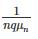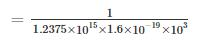= 5.05 Ω – cm

QUESTION: 10

Holes are injected into n-type Ge so that the at the surface of the semiconductor hole concentration is 1014/cm3. If diffusion constant of a hole in Ge is 49cm2/sec and minority carrier lifetime is τp = 10-3 sec. Then the hole concentration Δp at a distance of 4mm from the surface is ______1014/cm3.

Solution: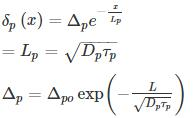= 1.6 × 1013/cm3

= 0.16 × 1014/cm3